Dr. J's Maths.com
Where the techniques of Maths
are explained in simple terms.

Algebra - Indices and Powers - basic questions.
Test yourself 1 - Solutions.

On this page, we have solutions to the expressions in the test aimed to evaluate your understanding of the five basic concepts underlying this topic.

Many of the solutions are presented showing the actual operations on the exponents. Very often we can just do these in our head - as long as they are easy to YOU - and avoid losing time. Instead of writing 1 + 3 but writing 4 instead, you save time. Any teacher will accept the 4 as being correct. If you do not write a 4, your teacher knows where you have made an error (e.g. a 3 indicates you multiplied). It is always a balance of time versus being careful. Do not overdo one of these actions at the expense of the other.

Multiplication of Powers:

Remember, by the First Law, we add the exponents attached to the same base.

 1. 3a × 2b × 5c × 4d = 120 abcd 2. y3 × y5 = y(3+5) = y8 3. 62 × 6-5 = 6(2-5) = 6-3 4. 2k3 × 3k4 = 6k(3+4) = 6k7 5. 3x2y × 5xy3 = 15 x(2+1) y(1+3)=15x3y4 6. 7-2 × 75 × 7-2 = 7(-2+5-2) = 71 = 7 7.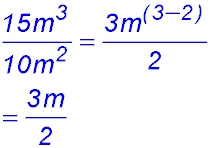. 8.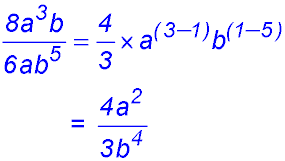. 9. 10x10 ÷ 5x6 = 2x(10-6) = 2x4 10.Comment.In a question with mutiple numbers and pronumerals, start at the left and collect all exponents for like terms. Then simplify. 11.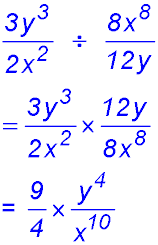. 12. 3j2 × 2k3m2 × 3jkmn2 = 18j(2+1) k(3+1) m(2+1) n2 = 18j3k4m3n2

Powers of powers:

 13. (k3)5 = k3×5 = k15 14. (c-3)-2 = c(-3×-2) = c6 15. (x-½)-2 = x(-½)(-2) = x1 = x 16. ((d2)-3)-1 = (d(2×-3))-1 = d(-6×-1) = d6 17. (3x2y3z)4 = 34x(2×4)y(3×4)z4 = 81x8y12z4 18. (2m3)3 × 4m5 = 23m(3×3)×4m5 = 32m(9+5) = 32m14

Negative powers:

 19.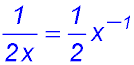20.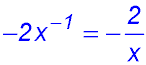. 21. (3-1)2 × 27 = 3-2 × 33 = 31 = 3 22.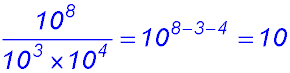23.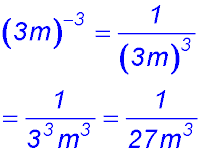24.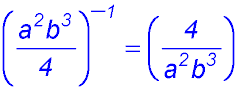Exponents of 1 or zero:

 25. 50 + 101 = 1 + 10 = 11 26. -(4m)0 = -1 27. 4a0 - 7b0 = 4 - 7 = -3 28. (33)0 × 52 = 1 × 25 = 25 29. 8m0 ÷ (2m)0 = 8 ÷ 1 = 8 30. (2x3 - 4y2)1 = 2x3 - 4y2

Fractional indices:

 31.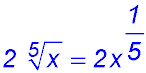. 32.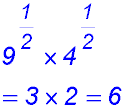33.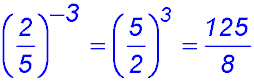34.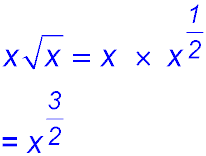35.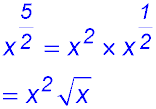. 36.Mixtures:

 37. (3t)2 × 4t3 = 9 t2 × 4 t3 = 36 t5 38.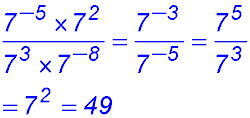39.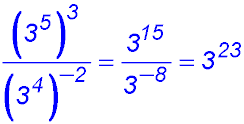40. 15m4n5 ÷ 5m2n4 = 3 m(4-2) n(5-4) = 3 m2 n 41. (3g3)3 ÷ (g2)4 =33g9 ÷ g8 = 27g 42. (10x2y-2)3 ÷ (2x-1y3)2 = (103 x6 y-6) ÷ (22 x-2y6) = 250 x(6+2) y(-6-6) = 250 x8 y-12